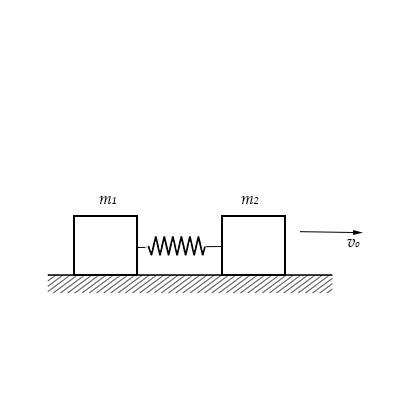# Two blocks of masses m_1 and m_2 are connected by a spring of spring constant k. The block of...

## Question:

Two blocks of masses {eq}m_1 {/eq} and {eq}m_2 {/eq} are connected by a spring of spring constant {eq}k {/eq}. The block of mass {eq}m_2 {/eq} is given a sharp impulse so that it acquires a velocity {eq}v_o {/eq} towards right. Find:

a) The velocity of the center of mass,

b) The maximum elongation that the spring will suffer.## Velocity:

The estimating of the value of distance that is completed by any object in an appropriate direction is termed as the velocity. The velocity of any particle can be determined in meter second inverse.

Given Data

• The mass of the first block is {eq}\left( {{m_1}} \right) {/eq}
• The mass of second block is {eq}\left( {{m_2}} \right) {/eq}
• The spring constant of the spring is {eq}\left( k \right) {/eq}
• The velocity of first block is {eq}\left( {{v_a}} \right) = 0 {/eq}
• The velocity of second block is {eq}\left( {{v_o}} \right) {/eq}

a) The expression for the velocity at the centre of mass is,

{eq}{v_{COM}} = \left( {\dfrac{{{m_1}{v_a} + {m_2}{v_b}}}{{{m_1} + {m_2}}}} \right) {/eq}

Substitute the values in the above equation.

{eq}\begin{align*} {v_{COM}} &= \left( {\dfrac{{{m_1} \times 0 + {m_2}{v_0}}}{{{m_a} + {m_b}}}} \right)\\ {v_{COM}} &= \left( {\dfrac{{{m_2}{v_0}}}{{{m_1} + {m_2}}}} \right) \end{align*} {/eq}

This is the required value of the velocity at the centre of mass.

b)

The condition for the maximum value of the elongation is,

{eq}{v_a} = {v_b} = {v_{COM}} {/eq}

The expression obtained by the application of the conservation law,

{eq}\dfrac{1}{2}{m_2}v_0^2 - \dfrac{1}{2}\left( {{m_1} + {m_2}} \right){\left( {\dfrac{{{m_2}{v_0}}}{{{m_1} + {m_2}}}} \right)^2} - \dfrac{1}{2}k{x^2} = 0 {/eq}

The expression can be further expressed as,

{eq}\begin{align*} \dfrac{1}{2}{m_2}v_0^2 - \dfrac{1}{2}\left( {{m_1} + {m_2}} \right)\left( {\dfrac{{{{\left( {{m_2}{v_0}} \right)}^2}}}{{{{\left( {{m_1} + {m_2}} \right)}^2}}}} \right) - \dfrac{1}{2}k{x^2} = 0\\ \dfrac{1}{2}{m_2}v_0^2 - \dfrac{1}{2}\left( {\dfrac{{\left( {{m_1} + {m_2}} \right){{\left( {{m_2}{v_0}} \right)}^2}}}{{{{\left( {{m_1} + {m_2}} \right)}^2}}}} \right) - \dfrac{1}{2}k{x^2} = 0\\ \dfrac{1}{2}{m_2}v_0^2 - \dfrac{1}{2}\left( {\dfrac{{{{\left( {{m_2}{v_0}} \right)}^2}}}{{\left( {{m_1} + {m_2}} \right)}}} \right) - \dfrac{1}{2}k{x^2} = 0\\ {m_b}v_0^2 - \left( {\dfrac{{{{\left( {{m_b}{v_0}} \right)}^2}}}{{\left( {{m_a} + {m_b}} \right)}}} \right) - k{x^2} = 0\\ {m_2}v_0^2\left( {1 - \dfrac{{\left( {{m_2}} \right)}}{{\left( {{m_1} + {m_2}} \right)}}} \right) - k{x^2} = 0 \end{align*} {/eq}

For the value of the elongation,

{eq}\begin{align*} {m_2}v_0^2\left( {1 - \dfrac{{\left( {{m_2}} \right)}}{{\left( {{m_1} + {m_2}} \right)}}} \right) = {kx^2}\\ {m_2}v_0^2\left( {1 - \dfrac{{\left( {{m_2}} \right)}}{{k\left( {{m_1} + {m_2}} \right)}}} \right) = {x^2}\\ \sqrt {{m_2}v_0^2\left( {1 - \dfrac{{\left( {{m_2}} \right)}}{{k\left( {{m_1} + {m_2}} \right)}}} \right)} = x\\ \sqrt {{m_2}\left( {1 - \dfrac{{\left( {{m_2}} \right)}}{{k\left( {{m_1} + {m_2}} \right)}}} \right)} \times {v_0} = x \end{align*} {/eq}

This is the required value of the elongation.